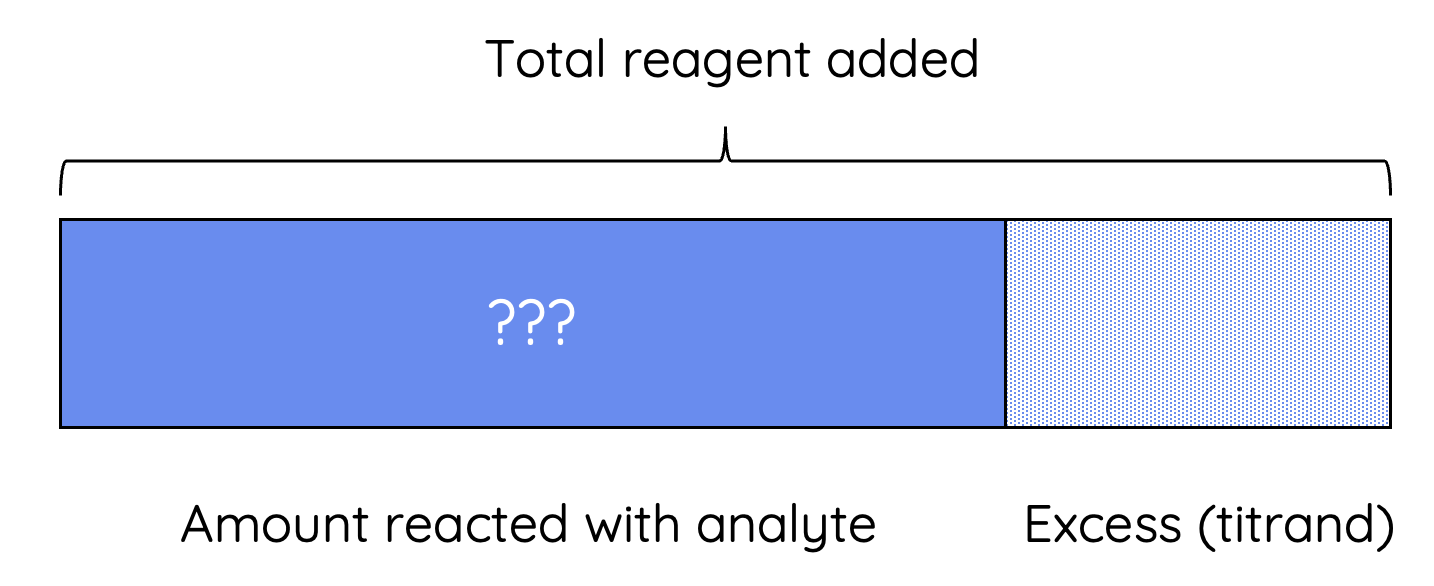# HSC Chemistry – Back Titration

This is part of the HSC Chemistry course under the topic Quantitative Analysis.

### HSC Chemistry Syllabus

• Conduct practical investigations to analyse the concentration of an unknown acid or base by titration

### What is Back Titration?

Back titration is a particular variant of titration that involves the determination of a solution's concentration by reacting it with an excess quantity of a standard solution.

This video discusses why back titration is performed and calculation problems involving back titration.

The amount in excess (left-over) is known as the titrand

The exact amount of titrand is then determined by titrating it with another standard solution.### Why do we do back titration?

There are numerous reasons why back titration is performed instead of direct titration.

• Back titration is used when direct titration is not possible or inaccurate An example of this is the use of an acid standard solution to determine the quantity of a sample of metal or metal carbonate
• Titration involving volatile substance e.g. ammonia
• Titration involving a slow reaction reaction where the equivalence point (when acid and base are added in their stoichiometric ratio to reach complete neutralisation) occurs before endpoint (when indicator changes colour)
• Titration involving weak acid and weak base where its equivalence point is difficult to determine using an indicator

### Examples of Back Titration Questions

Example 1

A 1.32 g sample of impure magnesium was analysed by allowing it to react with 100.0 mL of 0.750 mol/L HCl(aq). The remaining solution is titrated with 0.250 mol/L NaOH(aq), and the titre volume is 45.0 mL.

Assuming the impurities do not react with the acid, what is the percentage by mass of magnesium in the sample?

Watch video for step by step solutions

Example 2

A sample containing ammonium chloride was warmed with 150.0 mL of 1.00 mol/L sodium hydroxide. After all the ammonia had been driven off, the excess sodium hydroxide required 50.00 mL of 0.150 mol/L sulfuric acid for neutralisation.

What mass of ammonium chloride did the sample contain?

Watch video for step by step solutions